Previous: greenDyMSolver – transient, cavitation Up: TCFD Solvers Next: Incompressible Mathematical Model
This is an automatically generated documentation by LaTeX2HTML utility. In case of any issue, please, contact us at info@cfdsupport.com.

## Compressible Mathematical Model

The computational model solves following system of equations: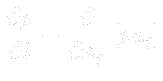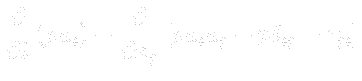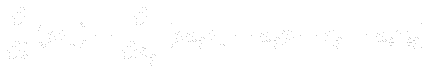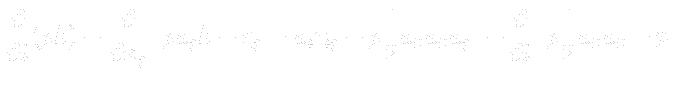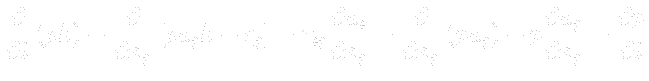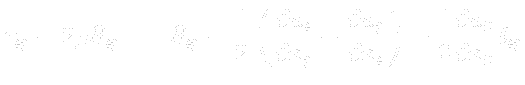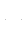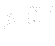• Mass conservation• Momentum conservation• Energy conservationand, two options
• where: Einstein summation is used,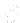is partial derivative,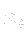is i-th Cartesian coordinate,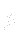is density,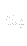is i-th velocity vector component,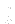is time,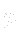is static pressure,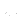shear stress tensor,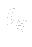is Kronecker delta,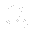is total specific energy,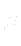is dynamic viscosity,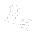is rate-of-deformation tensor,is static temperature,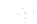is Prandtl number,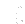specific gas constant,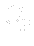specific heat capacity (at constant pressure),specific heat capacity (at constant volume),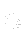i-th heat flux component (Fourier law),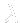heat conductivity coefficient.
• The whole system is closed with boundary conditions.